# Substitution Theorem

As name implies, the main concept of this theorem which is based upon substitution of one element by another equivalent element. Substitution theorem gives us some special insights in circuit behavior. This theorem is also used to prove several other theorems.

## Statement of Substitution Theorem

Substitution theorem states that if an element in a network is replaced by a voltage source whose voltage at any instant of time is equals to the voltage across the element in the previous network then the initial condition in the rest of the network will be unaltered or alternately if an element in a network is replaced by a current source whose current at any instant of time is equal to the current through the element in the previous network then the initial condition in the rest of the network will be unaltered.

## Explanation of Substitution Theorem

Let us take a circuit as shown in fig – a,Let, V is supplied voltage and Z1, Z2 and Z3 is different circuit impedances. V1, V2 and V3 are the voltages across Z1, Z2 and Z3 impedance respectively and I is the supplied current whose I1 part is flowing through the Z1 impedance whereas I2 part is flowing through the Z2 and Z3 impedance.

Now if we replace Z3 impedance with V3 voltage source as shown in fig-b or with I2 current source as shown in fig-c then according to Substitution Theorem all initial condition through other impedances and source will remain unchanged.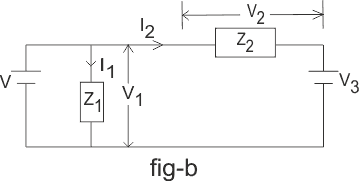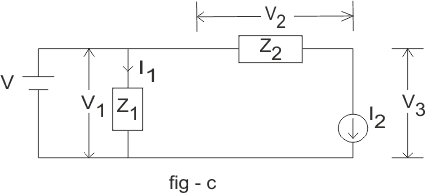i.e. – current through source will be I, voltage across Z1 impedance will be V1, current through Z2 will be I2 etc.

### Example of Substitution Theorem

For more efficient and clear understanding let us go through a simple practical example:
Let us take a circuit as shown in fig – d.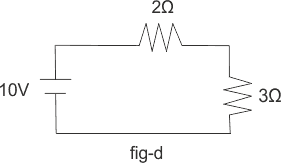As per voltage division rule voltage across 3Ω and 2Ω resistance areIf we replace the 3Ω resistance with a voltage source of 6 V as shown in fig – e, then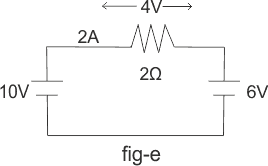According to Ohm’s law the voltage across 2Ω resistance and current through the circuit is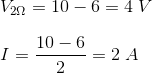Alternately if we replace 3Ω resistance with a current source of 2A as shown in fig – f, then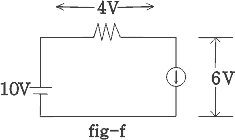Voltage across 2Ω is V = 10 – 3× 2 = 4 V and voltage across 2A current source is V2A = 10 – 4 = 6 V
We can see the voltage across 2Ω resistance and current through the circuit is unaltered i.e all initial condition of the circuit is intact.

Want To Learn Faster? 🎓
Get electrical articles delivered to your inbox every week.
No credit card required—it’s 100% free.